# Electromagnetic Waves Questions

## Multiple Choice Questions

Question 1
What is the frequency range of microwaves?
(a) $10^9$ Hz to $10^{12}$ Hz
(b) $10^{14}$ Hz to $10^{16}$ Hz
(c) $10^{4}$ Hz to $10^{6}$ Hz
(d) $10^{2}$ Hz to $10^{4}$ Hz

(a) is correct

Question 2
If E and B represent electric and magnetic field vectors of the electromagnetic wave, the direction of propagation of electromagnetic wave is along
(a) E.
(b) B.
(c) B X E.
(d) E X B.

(d)

Question 3
A charged particle oscillates about its mean equilibrium position with a frequency of $10^9$ Hz. The electromagnetic waves produced
(a) will have frequency of $10^9$ Hz.
(b) will have frequency of $2 \times 10^9$ Hz.
(c) will have a wavelength of 0.3 m.
(d) fall in the region of radiowaves

(a),(c) and (d)

Question 4
The correct ascending order for the frequencies of below electromagnetic radiation is
(c) Gamma Rays, Radiowave , Microwave,X-rays,
(d) Radiowave , Microwave,X-rays, Gamma Rays

(d) is correct option

Question 5
Speed of E.M wave is free space is given
(A) $c=\frac {1}{\sqrt {\mu _0 \epsilon _0}}$
(B) $c=\frac {\sqrt {\epsilon _0}}{\sqrt {\mu _0 }}$
(C) $c=\frac {\sqrt { \mu _0}}{\sqrt {\epsilon _0 }}$
(D) $c=\frac {1}{\mu _0 \epsilon _0}$

(a)

Question 6
which of these electromagnetic spectrum does the wavelength $10^{-10}$ m correspond to?
(a)Gamma Rays
(b)X-rays
(d) Microwave

(b)

Question 7
A plane electromagnetic wave propagating along x direction can have the following pairs of E and B
(a) $E_x$, $B_y$
(b) $E_y$, $B_z$
(c) $B_x$, $E_y$
(d) $E_z$, $B_y$

(b) and (d) as E and B will be perpendicular to the direcition of propagation and also perpendicular to each other

Question 8
The source of electromagnetic waves can be a charge
(a) moving with a constant velocity.
(b) moving in a circular orbit.
(c) at rest.
(d) falling in an electric field.

(b) and (d)

Question 9
Match the column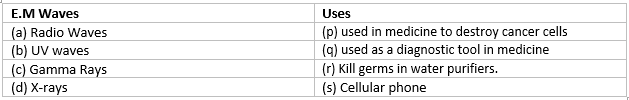(a) a -> s, b -> r , c -> p,d -> q.
(b) a -> s, b -> r , c -> q,d -> p.
(c) a -> r, b -> s , c -> p,d -> q.
(d) a -> p, b -> q , c -> s,d -> r

(a) is the correct choice

Question 10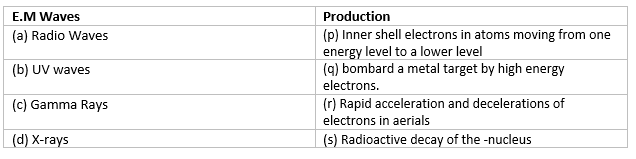(a) a -> p, b -> r , c -> s,d -> q.
(b) a -> q, b -> r , c -> s,d -> p.
(c) a -> r, b -> p , c -> s,d -> q.
(d) a -> p, b -> q , c -> s,d -> r

(c) is the correct option

Question 11
Propagation constant of the E.M wave is given by
(a) $\frac {2 \pi}{\omega}$
(b) $\frac {c}{\omega}$
(c) $\frac {2 \pi}{c}$
(d) $\frac {\omega}{c}$

Propagtion constant $k = \frac {2 \pi}{\lambda } = \frac {\omega}{c}$ hence (d) is the correct option

Question 12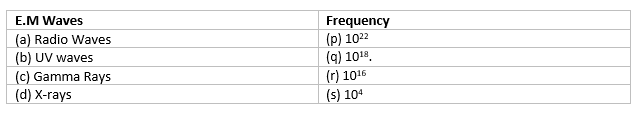(a) a -> p, b -> r , c -> s,d -> q.
(b) a -> s, b -> r , c -> p,d -> q.
(c) a -> r, b -> p , c -> s,d -> q.
(d) a -> p, b -> q , c -> s,d -> r

(b) is correct

Question 13
A linearly polarized electromagnetic wave given as $\vec{E}=E_0 \cos (kz - \omega t)\vec{i}$ is incident normally on a perfectly reflecting infinite wall at z = a. Assuming that the material of the wall is optically inactive, the reflected wave will be given as
(a) $\vec{E_r}=E_0 \cos (kz + \omega t)\vec{i}$
(b) $\vec{E_r}=-E_0 \cos (kz + \omega t)\vec{i}$
(c) $\vec{E_r}=-E_0 \cos (kz - \omega t)\vec{i}$
(d) $\vec{E_r}=E_0 \sin (kz - \omega t)\vec{i}$

When a wave is reflected from perfectly reflecting infinite wall at z = a, the reflected wave is without change in type of wave but with a change in phase by $\pi$ radian. Therefore, for the reflected wave we use z=-z,$\vec{i}=-\vec{i}$
The reflected em wave is
$\vec{E_r}=E_0 \cos (k(-z) + \omega t + \pi)(-\vec{i})$
or
$\vec{E_r}=E_0 \cos (kz + \omega t)\vec{i}$

Question 14
The ratio between amplitude of magnetic field and electric field of a E.M wave in free space is given by ?
(a) $1 : c$
(b) $c : 1$
(c) $c^2 : 1$
(d) $1 : c^2$

(a) is correct

Question 15
Name the part of the electromagnetic spectrum which is used in green house to keep plants warm
(a) X rays.
(b) UV rays.
(c) infrared.
(d) Micro-wave.

(c)

Question 16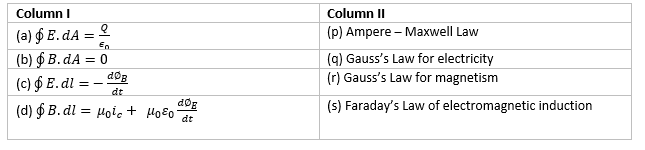(a) a -> q, b -> r , c -> s,d -> p.
(b) a -> s, b -> r , c -> p,d -> q.
(c) a -> r, b -> p , c -> s,d -> q.
(d) a -> p, b -> q , c -> s,d -> r

(a) is correct

Question 17
Which of the following electromagnetic rays has maximum wavelength
(a) UV rays
(b) Infrared rays
(c) X -rays

(d) is correct

Question 18
A plane E.M wave of frequency 30MHz travels in the free space along x-direction.The electric field component of the wave at a particular point in space and time is E= 6 V/m along y -direction.Its magnetic field component B at this point would be
(a) $2 \times 10^{-8}$ T along y-direction
(b) $6 \times 10^{-8}$ T along y-direction
(c) $6 \times 10^{-8}$ T along z-direction
(d) $2 \times 10^{-8}$ T along z-direction

$\frac {E}{B} = c$
Therefore
B= $2 \times 10^{-8}$ T
To find the direction, we note that E is along y-direction and the wave propagates along x-axis. Therefore, B should be in a direction perpendicular to both x- and y-axes. Therefore B is along the z-direction.
hence (d) is correct

Question 19
Dimension of $\frac {1}{\mu _0 \epsilon _0}$ where symbols have their usual meaning is
(a) $[L^2T^{-2}]$
(b) $[L^2T^{2}]$
(c) $[L^{-1}T]$
(d) $[LT^{-1}]$

(a)
as
$c= \frac {1}{\sqrt {\mu _0 \epsilon _0}}$

Question 20
The pressure exerted by an electromagnetic wave of intensity I (Wm-2) on a non-reflecting surface os (c is the velocity of light)
(a) $Ic$
(b) $Ic^2$
(c) $\frac {I}{c^2}$
(d) $\frac {I}{c}$

(d)

## Text Based Questions(long)

Question 1
How is the electromagnetic wave produced?. Draw a sketch of a plane E.M wave propagting along X-axis depicting the direction of oscillating electric and magentic fields

Question 2
Identify the E.M waves whose wavelength lie in the range (a) $10^{-11} \; m < \lambda < 10^{-4} \; m$
(b) $10^{-4} \; m < \lambda < 10^{-6} \; m$
(c) $10^{-12} \; m < \lambda < 10^{-8} \; m$
(d)$10^{-3} \; m < \lambda < 10^{-1} \; m$
Write one use of each of those

(a)Gamma rays. They are used in treatment of cancer and tumor . They are also used in nuclear reaction
(b)Infrared . They are used in green house to keep plantrs warm and they are used in physiotherapy
(c) X-rays . This is used in surgery and detective department

Question 3
How does Ampere-Maxwell law explain the flow of current through a capacitor, when it is being charged by a battery? Write the expression for the displacement current in terms of the rate of change of electric flux .

When we charge a capacitor with the help of a cell, current flows all through the circuit except the part of circuit which lies between two plates of capacitor. There is no current found in that gap. But it was found that an electric field exist inside the plates therefore, indicating existance of magnetic field which leads to inconsistency in the Ampere s circuital law. This was found by Maxwell's and He suggested the existence of an additional current, called displacement current, to remove this inconsistency. This displacement current is due to time-varying electric field and is given by

$i_d= \epsilon _0 \frac {d \phi _E}{dt}$

Question 4
Write Maxwell's generalization of Ampere's Circuital Law?

Question 5
Name the parts of the electromagnetic spectrum which is
(b) used to treat muscular strain
(c) used as a diagnostic tool in medicine
Write in brief, how these waves can be produced

a. Microwaves are suitable for radar systems that are used in aircraft navigation.
These rays are produced by special vacuum tubes, namely Klystrons, magnetrons and Gunn diodes.
b. Infrared waves are used to treat muscular strain.
These rays are produced by hot bodies and molecules.
c. X rays are used as a diagnostic tool in medicine.
These rays are produced when high energy electrons are stopped suddenly on a metal of high atomic number.

Question 1
For a plane electromagnetic wave, propagating along the z-axis, write the two possible pair of its oscillating electric and magnetic fields. How are the peak values of these (oscillating) fields related to each other?

$\vec{E}= E_x \vec{i}=E_0 \sin (kz - \omega t)\vec{i}$
$\vec{B}= B_y \vec{j}=B_0 \sin (kz - \omega t)\vec{j}$
$\frac {E_0}{B_}0=c$ = velocity of electromagnetic wave.

Question 2
(a) If the earth did not have atmosphere, would its average surface temperature be higher or lower than what it is now ? Explain.
(b) An EM wave exerts pressure on the surface on which it is incident. Justify..?

(a) Average surface temperature will be lower. Because there will be no green house effect in absence of atmosphere.
(b)Since electromagnetic waves carry both energy and momentum, therefore, they exert pressure on the surface on which they are incident.

Question 3
Question 4
Write an expression fotr the momentum carrried by an E.M wave?

An electromagnetic waves transport linear momementum as it travels through the space. If U is the total energy of electromagnetic waves transfer to the surface in time t,then total linear momentum delivered to the surface is
$p = \frac {U}{c}$
The above was the momentum transferred in case of complete absorption of light
If the wave is totally reflected , the momentum delivered will be
$p = \frac {2U}{c}$

Question 5
What is green house effect?
Question 6
Electromagnetic waves with wavelength
(i) $\lambda _1$ is used in satellite communication.
(ii) $\lambda _2$ is used to kill germs in water purifies.
(iii) $\lambda _3$ is used to detect leakage of oil in underground pipelines.
(iv)$\lambda _4$ is used to improve visibility in runways during fog and mist conditions.
(a) Identify and name the part of electromagnetic spectrum to which these radiations belong.
(b) Arrange these wavelengths in ascending order of their magnitude.
(c) Write one more application of each

(a) $\lambda _1$ -> Microwave
$\lambda _2$ -> UV
$\lambda _3$ -> X rays
$\lambda _4$ -> Infrared
(b) $\lambda _3$ < $\lambda _2$ < $\lambda _4$ < $\lambda _1$
UV - LASIK eye surgery
X-ray - Bone fracture identification (bone scanning)
Infrared - Optical communication.

## Numerical Questions

Question 1
The oscillating electric field of an electromagnetic wave is given by
$E_Y = 30 \sin (2 \times 10^{11} t + 300 \pi x)$ V/m
(i) Find the direction of propagation of wave
(ii)Find the wavelength of the wave
(iii) write down the expression for magnetic field?

(i) The direction of propagation of wave is along negative x axis
(i) $E_Y = 30 \sin (2 \times 10^{11} t + 300 \pi x)$ V/m
Standard Equation is
$E_Y = E_0 \sin [2 \pi ( \frac {x}{\lambda} + \frac {t}{T})]$ V/m
On comparing
$E_0=30$ V/m and $\frac {2 \pi}{\lambda } = 300 \pi$
or
$\lambda = \frac {1}{150} =.67$ cm
(ii) Now
$B_0 = \frac {E_0}{c} = 10^{-7}$ T
The magnetic field is perpendicular to the direction of propagation i,e x -axis and direction to electric field (y -axis).So direction of magnetic field will be along z direction and expression will be given by
$B_z= 10^{-7} \sin (2 \times 10^{11} t + 300 \pi x)$ T

Question 2
A plane electromagnetic wave of frequency 25 MHz travels in free space along the x-direction. At a particular point in space and time, $\vec{E}=6 \vec{j}$ V/m. What is B at this point?

Now
$B_0 = \frac {E_0}{c} = 2 \times 10^{-8}$ T
To find the direction, we note that E is along y-direction and the wave propagates along x-axis. Therefore, B should be in a direction perpendicular to both x- and y-axes. Using vector algebra, E X B should be along x-direction. Since, $(+ \vec{j}) (\vec{k} ) = \vec{i}$ , B is along the z-direction. Thus, $B = 2 \times 10^{ 8} \vec{k}$ T

Question 3
An electromagnetic wave is travelling in medium with velocity $v=v\vec{i}$ the electric field oscillations of this wave are along y axis
(i) identify the direction in which the magnetic field oscillations are taking place in the e.m. wave
(ii) how are magnitude of elctric and magnetic fields in the electromagnetic wave related to each other?

(i) To find the direction, we note that E is along y-direction and the wave propagates along x-axis. Therefore, B should be in a direction perpendicular to both x- and y-axes. Using vector algebra, E X B should be along x-direction. Since, $(+ \vec{j}) (\vec{k} ) = \vec{i}$ , B is along the z-direction.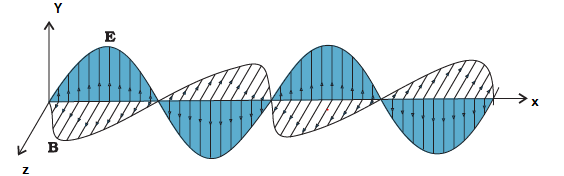(ii) $\frac {E_0}{B_0} =c$

Question 4
A capacitor, made of two parallel plates each of plate area A and separation d, is being charged by an external ac source. Show that the displacement current inside the capacitor is the same as the current charging the capacitor.

Electric Field inside the capacitor is given by
$E= \frac {q}{\epsilon _0 A}$
Where q is the charge accumulated over the plate.
Electric Flux through the plate is
$\phi _E = EA = \frac {q}{\epsilon _0}$
Displacement current is given by
$I_D= {\epsilon _0} \frac {d\phi _E}{dt} = \frac {dq}{dt}$
But $\frac {dq}{dt}$ is the rate at which charge flows to positive through the conducting wire
Hence $I_D=I_C$

Question 5
In a plane E.M wave ,the electric field oscillates sinusoidally at a frequency of $2 \times 10^{10}$ Hz and amplitude 48 V/m
(a) what is the wavelength of a wave?
(b) What is the amplitude of the oscillating magnetic field
(c) show that averge energy density of the $\vec{E}$ field equals the average energy density of the $\vec{B}$ field

(a)Wavelength is given by
$\lambda = \frac {c}{\mu} = \frac {3 \times 10^8}{2 \times 10^{10} = 1.5 \times 10^{-2}$ m
(b)
$B_0 = \frac {E_0}{c} = \frac {48}{3 \times 10^8} = 1.6 \times 10^{-7}$ T
(c) Average energy density of $\vec{E}$ is given by
$u_E= \frac {1}{4} \epsilon _0 E_0^2$
Average energy density of $\vec{B}$ is given by
$u_B= \frac {1}{4 \mu _0} B_0^2$
Now
$E_0= cB_0$ or $c^2= \frac {1}{\mu _0 \epsilon _0}$
Therefore
$u_E= \frac {1}{4} \epsilon _0 E_0^2 = \frac {1}{4} \epsilon _0 c^2 B_0^2= \frac {1}{4} .\epsilon _0 . \frac {1}{\mu _0 \epsilon _0} . B_0^2 = \frac {1}{4 \mu _0} B_0^2 = u_B$